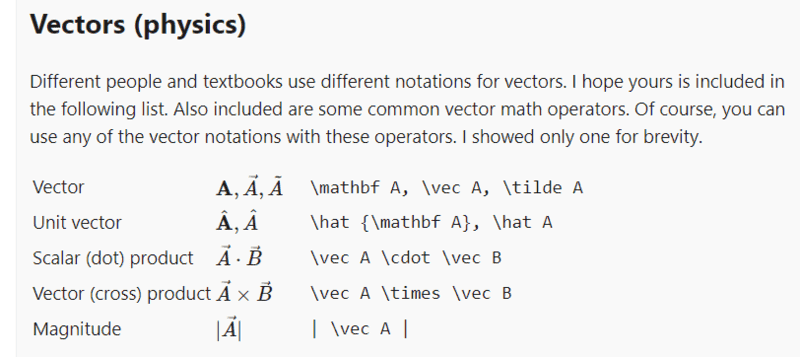# Confusion about the angle between two vectors in a cross product

• B
• tbn032
The angle between vector A⃗ and vector B⃗ can be measured in two ways, clockwise and anti-clockwise. The clockwise wise measurement is generally taken to be negative and the anti-clockwise wise measurement is generally taken to be positive.When looking at the cross product, the angle between the two vectors is always taken into consideration. However, the angle between the vectors can be measured in two ways, clockwise and anti-clockwise. The clockwise way of measuring the angle is generally taken to be negative and the anti-clockwise way of measuringf

#### tbn032

The magnitude of cross product is defined of vector A⃗ and B⃗ as |A⃗×B⃗|=|A⃗||B⃗|sinθ where θ is defined as the angle between the two vector and 0≤θ≤π.the domain of θ is defined 0≤θ≤π so that the value of sinθ remains positive and thus the value of the magnitude |A⃗||B⃗|sinθ also remain positive (magnitude cannot be negative).

But if A⃗=1i^ and B⃗ =-j^ then the angle between these vector would be -π/2 but the magnitude of |A⃗×B⃗|=|A⃗||B⃗|sinθ where the domain of θ is defined 0≤θ≤π.

how can this angle(-π/2) be incorporated in the formula so that the magnitude of cross product of these vectors could be found. In general, how can the θ whose value is π≤θ≤2π incorporated in the formula so that the magnitude of the cross product of the vectors can be calculated.

The angle between vector A⃗ and B⃗ can be θ=π/2 if we measure anti-clockwise (From vector B⃗ to A⃗ and θ=-π/2 if we measure the angle clockwise(from vector A⃗ to B⃗).how can I say which angle to pick.

Last edited by a moderator:
The magnitude of cross product is defined of vector A⃗ and B⃗ as |A⃗×B⃗|=|A⃗||B⃗|sinθ where θ is defined as the angle between the two vector and 0≤θ≤π.the domain of θ is defined 0≤θ≤π so that the value of sinθ remains positive and thus the value of the magnitude |A⃗||B⃗|sinθ also remain positive (magnitude cannot be negative).

But if A⃗=1i^ and B⃗ =-j^ then the angle between these vector would be -π/2 but the magnitude of |A⃗×B⃗|=|A⃗||B⃗|sinθ where the domain of θ is defined 0≤θ≤π.

how can this angle(-π/2) be incorporated in the formula so that the magnitude of cross product of these vectors could be found. In general, how can the θ whose value is π≤θ≤2π incorporated in the formula so that the magnitude of the cross product of the vectors can be calculated.

The angle between vector A⃗ and B⃗ can be θ=π/2 if we measure anti-clockwise (From vector B⃗ to A⃗ and θ=-π/2 if we measure the angle clockwise(from vector A⃗ to B⃗).how can I say which angle to pick.
We have ##\vec A \times \vec B = |A||B|\sin \theta##, hence ##|\vec A \times \vec B| = |A||B||\sin \theta|##. Or, when dealing with the modulus you could change your definition of ##\theta## to lie in the range ##[0, \pi)##.

The formula shown here (https://en.wikipedia.org/wiki/Cross_product, in the Properties section) gives the magnitude of the cross product as $$||\vec a \times \vec b|| = ||\vec a|| ||\vec b|| |\sin(\theta)|$$

BTW, in what you wrote there are some extra symbols whose purpose I don't understand.

But if A⃗=1i^ and B⃗ =-j^
What is the meaning of the square after A?
What is the meaning of the caret after j?

BTW, in what you wrote there are some extra symbols whose purpose I don't understand.

What is the meaning of the square after A?
What is the meaning of the caret after j?
I just meant i cap and j cap (unit vectors along x-axis and y-axis, respectively)

Or, when dealing with the modulus you could change your definition of ##\theta## to lie in the range ##[0, \pi)##.
I think the theta will lie in the range ##[0, \pi]## instead of ##[0, \pi)##.

I just meant i cap and j cap (unit vectors along x-axis and y-axis, respectively)••topsquark and dlgoff
Note that the cross-product is often associated with the oriented parallelogram formed by its factors. (It might help to think "bi-vector. https://en.wikipedia.org/wiki/Bivector )
The magnitude of the cross-product is equal to the area of that parallelogram.
With the tails of the vectors together, the interior angle is a signed-angle whose magnitude is not larger than ##\pi##.

The magnitude of the cross-product is equal to the area of that parallelogram.
With the tails of the vectors together, the interior angle is a signed-angle whose magnitude is not larger than ##\pi##.
In many of the definition of cross product, I have seen the ##\theta##(angle between the two vector) is in the range of 0≤##\theta##≤π.

The angle between the two vector can be measured in two ways, clockwise and anti-clockwise. The clockwise wise measurement is generally taken to be negative and the anti-clockwise wise measurement is generally taken to be positive.

How can the ##\theta## always lie in the 0≤##\theta##≤π.for example, take two vectors ##\vec A## =1##\hat i## and ##\vec B## =-1##\hat j##.the angle between these vectors could be measured -π/2 and π/2 if we measure it clockwise and anticlockwise respectively. How can the -π/2 incorporated in the range 0≤##\theta##≤π.

is it the case that we ignore clockwise measurement when measuring the angle between vector or is it the case that the ##\theta## which is present in the formula |A⃗×B⃗|=|A⃗||B⃗|sin##\theta## is just the magnitude of the angle present between the two vector and direction(clockwise or anti-clockwise) is not considered(##\theta##=|angle between the vectors|)

Last edited: